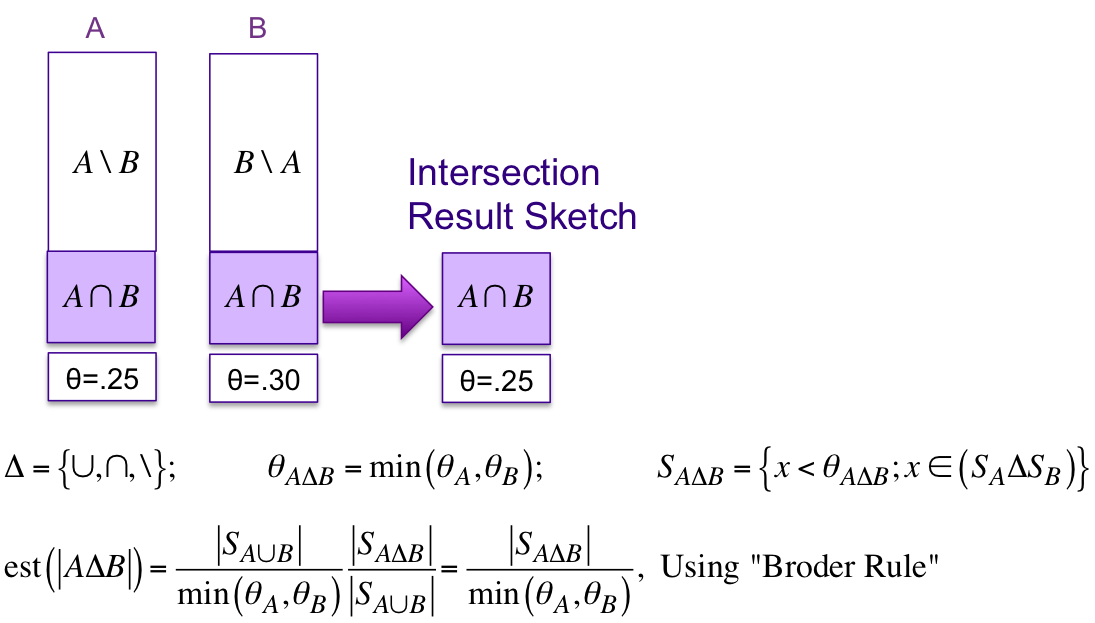## Theta Sketch Set Operations

The Theta Sketch definition enables a uniform and simplified approach to performing the three standard set operations, Union (∪), Intersection (∩) and Difference (\).

The diagram below illustrates how the Intersection operation can be performed by examining the internal hash values (entries) of both sketches, A and B. Note that the result of a simple 2-way set operation is another sketch! Performing Union and Difference operations are similar. The equations for all three set operations are generalized below where the delta symbol, Δ, represents one of the three set operations.The fact that set operations produce sketches as results enables full set expressions, such as
((A ∪ B) ∩ (C ∪ D))\(E ∪ F).

The Union and Intersection operations are symmetric (i.e., sketch order insensitive) and naturally iterative. The AnotB operation, however, is asymmetric (i.e., sketch order sensitive) and not iterative. For example:

int k = 4096;

for (int i=1;  i<=10; i++) { skA.update(i); }
for (int i=1;  i<=20; i++) { skB.update(i); }
for (int i=6;  i<=15; i++) { skC.update(i); } //overlapping set

Union union = Sketches.setOperationBuilder().setNominalEntries(k).buildUnion();
union.update(skA);
union.update(skB);
// ... continue to iterate on the input sketches to union

CompactSketch unionSk = union.getResult();   //the result union sketch
System.out.println("A U B      : "+unionSk.getEstimate());   //the estimate of union

//Intersection is similar

Intersection inter = Sketches.setOperationBuilder().setNominalEntries(k).buildIntersection();
inter.update(unionSk);
inter.update(skC);
// ... continue to iterate on the input sketches to intersect

CompactSketch interSk = inter.getResult();  //the result intersection sketch
System.out.println("(A U B) ^ C: "+interSk.getEstimate());  //the estimate of intersection

//The AnotB operation is a little different as it is stateless and not iterative:

AnotB aNotB = Sketches.setOperationBuilder().setNominalEntries(k).buildANotB();
aNotB.update(skA, skC);

CompactSketch not = aNotB.getResult();
System.out.println("A \\ C      : "+not.getEstimate()); //the estimate of AnotB

/* OUTPUT:
A U B      : 20.0
(A U B) ^ C: 10.0
A \ C      : 5.0
*/